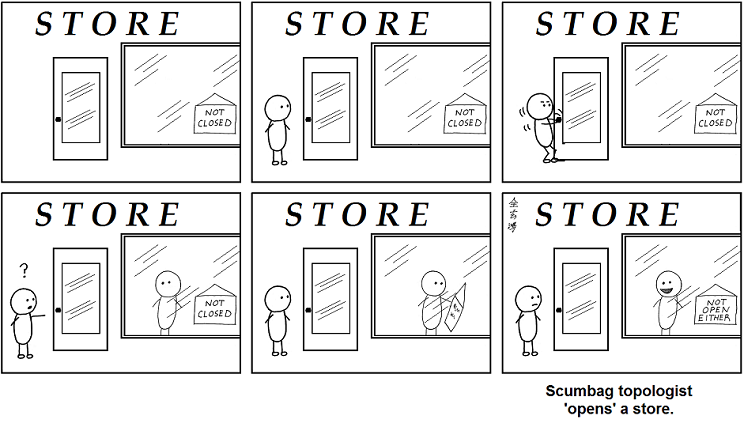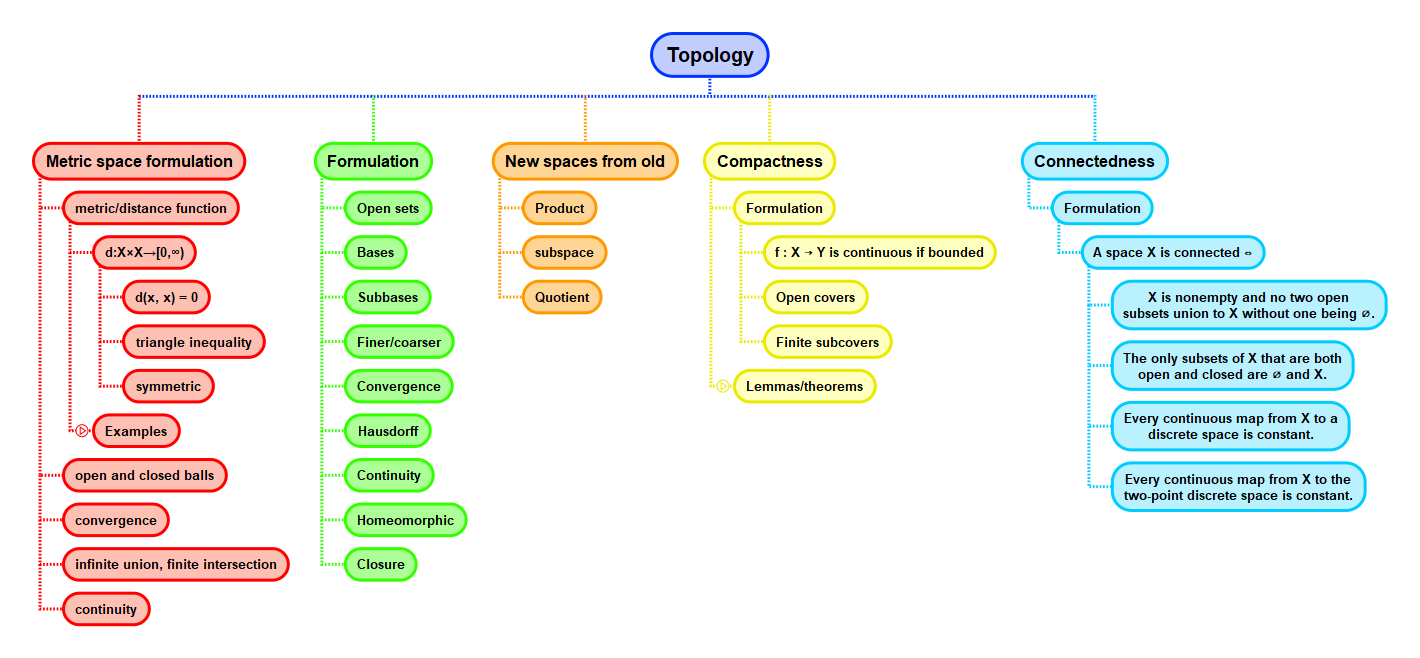Show Question
Math and science::Topology

# Metric space. Open and closed sets

### Open and closed subsets

Let $$X$$ be a metric space.

Open in $$X$$
A subset $$U$$ of $$X$$ is open in $$X$$ iff for all $$u \in U$$, there exists an $$\varepsilon > 0$$ such that $$B(u, \varepsilon) \subseteq U$$.
Closed in $$X$$
A subset $$V$$ of $$X$$ is closed in $$X$$ iff $$X \setminus V$$ is open in $$X$$.

Tom Leinster describes the openness of $$U$$:

Thus, $$U$$ is open if every point of $$U$$ has some elbow room—it can move a little bit in each direction without leaving $$U$$.

Personally, I like the phrase: every element of an open set has a neighbourhood.

### ε-balls

Open ε-balls are open, and closed ε-balls are closed. Consider trying to prove this. They are open on account of the definition of openness, not by their own definition alone, despite their names being suggestive.

### Open, closed, neither or both

Subsets of a metric space can be any combination of open and closed.### Open subset, closed subset

There's no such thing as an open set that is not an open subset; $$U$$ is open in $$X$$. The importance of this is that a set $$U$$ may be open in one space and not open in another. $$[0, 1)$$ is not open in $$\mathbb{R}$$ but is open in $$[0, 2]$$.

## Context#### Source

Tom Leinster's Topology notes

Comic: